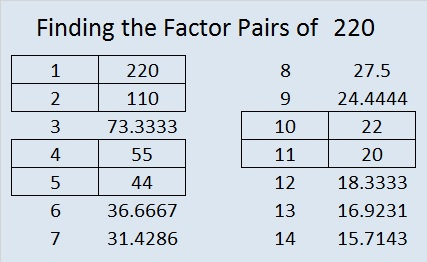# 220 and Level 2

• 220 is a composite number.
• Prime factorization: 220 = 2 x 2 x 5 x 11, which can be written (2^2) x 5 x 11
• The exponents in the prime factorization are 2, 1 and 1. Adding one to each and multiplying we get (2 +1)(1 + 1)(1 + 1) = 3 x 2 x 2 = 12. Therefore 220 has 12 factors.
• Factors of 220: 1, 2, 4, 5, 10, 11, 20, 22, 44, 55, 110, 220
• Factor pairs: 220 = 1 x 220, 2 x 110, 4 x 55, 5 x 44, 10 x 22,  or 11 x 20
• Taking the factor pair with the largest square number factor, we get √220 = (√4)( √55) = 2√55 ≈ 14.832Excel file of puzzles and previous week’s factor solutions: 10 Factors 2014-09-01This site uses Akismet to reduce spam. Learn how your comment data is processed.